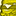# QlikView App Dev

Discussion Board for collaboration related to QlikView App Development.

Announcements
Action-Packed Learning Awaits! QlikWorld 2023. April 17 - 20 in Las Vegas: REGISTER NOW
cancel
Showing results for
Did you mean:Not applicable

## count today - 7 days

Hi allI have the following fields:

Date

Activity_id

I also have a table chart with the following expression: count(Activities_id).

I want to count (Activities_id) using set analysis, so that I'll count all the activities which were performed 7 days ago till today.

I'm not sure how I can do that... I'm using the following expression in order to count last week activities:

=count

(DISTINCT

{\$<[YearWeek_Num] = {\$(=MAX([YearWeek_Num])-1)}>}

[Activities_id]

)

thanks!

1 Solution

Accepted SolutionsContributor III

Hi Michal,

=

count({<Date={">=\$(=date(today()-7))<=\$(=date(today()))"}>} Activity_id)

11 RepliesPartner - Champion III

I should do:

count

(DISTINCT

{\$<[YearWeek_Num] = {\$(=(year(Today()-7)*100+week(today()-7)))}>}

[Activities_id]

)Employee

Assuming that the field names are Date and Activity_id, this will work:

count({<Date={">=date(today()-7)<=date(today()"}>} distinct Activity_id)Partner - Creator II

count({<Date={">=date(today()-7)<=date(today())"}>} distinct Activity_id)

U missed a BracketEmployee

thanksNot applicable
Author

Hi! thank you for your help.

for some reason the result is '0' although I have activities in the last 7 days

thanksContributor III

Hi Michal,

=

count({<Date={">=\$(=date(today()-7))<=\$(=date(today()))"}>} Activity_id)Employee

OK, here is a more reliable way:

=count({<Date={"\$(='>='&date(today()-7)&'<='&date(today()))"}>} distinct Activity_id)Specialist II

Hi,

Michal

Try this

=count({<Date={"=date(today()-7)"}>} distinct Activity_id)MVP

Hi

Try like this

count({<Date={">=\$(=date(today()-7))<=\$(=date(today()))"}>} distinct Activity_id)

Thanks & Regards,
Mayil Vahanan R